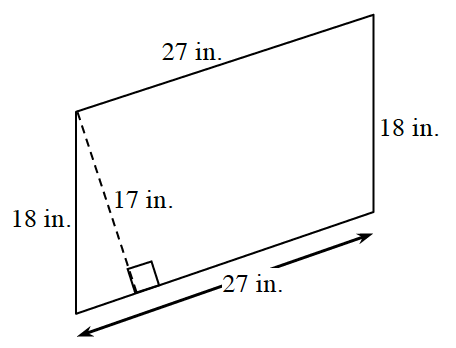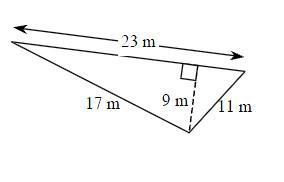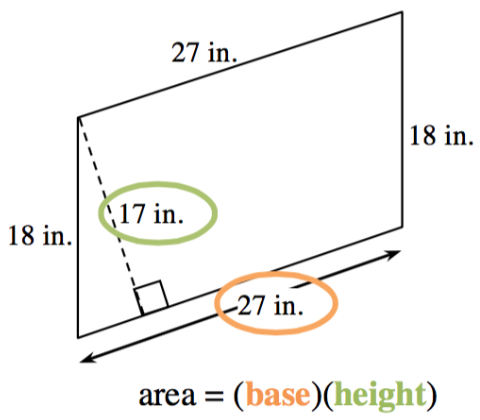### Home > MC2 > Chapter 3 > Lesson 3.2.1 > Problem3-60

3-60.

Find the area of each figure below.

1.1..

First, examine each shape to determine which type of shape it is. Look for characteristics such as right angles, parallel sides, and number of sides.

After you have determined the shape, recall the area formulas specific to that shape. In this case, a trapezoid and a triangle.

Try solving for the area of the parallelogram and triangle using these formulas.$459$ sq. in.